# Equipotential Surface – Properties, Example

0
161

## What is Equipotential Surface?

The surface, the locus of all points at the same potential, is known as the equipotential surface. No work is required to move a charge from one point to another on the equipotential surface. In other words, any surface with the same electric potential at every point is termed as an equipotential surface.

### Work Done in Equipotential Surface

The work done in moving a charge between two points in an equipotential surface is zero. If a point charge is moved from point VA to VB, in an equipotential surface, then the work done in moving the charge is given by

W = q0(VA –VB)

As VA – VB is equal to zero, the total work done is W = 0.

## Properties of Equipotential Surface

1. The electric field is always perpendicular to an equipotential surface.
2. Two equipotential surfaces can never intersect.
3. For a point charge, the equipotential surfaces are concentric spherical shells.
4. For a uniform electric field, the equipotential surfaces are planes normal to the x-axis
5. The direction of the equipotential surface is from high potential to low potential.
6. Inside a hollow charged spherical conductor, the potential is constant. This can be treated as equipotential volume. No work is required to move a charge from the centre to the surface.
7. For an isolated point charge, the equipotential surface is a sphere. i.e. concentric spheres around the point charge are different equipotential surfaces.
8. In a uniform electric field, any plane normal to the field direction is an equipotential surface.
9. The spacing between equipotential surfaces enables us to identify regions of a strong and weak field i.e. E= −dV/dr ⇒ E ∝ 1/dr

### Equipotential surfaces

• Surface over which the electric potential is same everywhere is called an equipotential surface.
• Equipotential surfaces are the graphical way to represent potential distribution in an electric field.
• We can draw equipotential surfaces through a space having the electric field.
• For a positive charge, the electric field would be in radially outward direction and the equipotential surfaces would be concentric spheres with centers at the charge as shown below in the figure.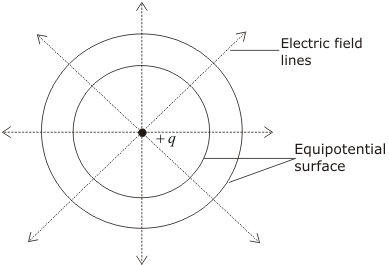• Since electric potential remains same everywhere on an equipotential surface from this it follows that PE of a charged body is same at all points on this surface. This shows that work done in moving a charged body between two points on an equipotential surface would be zero.
• At every point on the equipotential surface, electric field lines are perpendicular to the surface. This is because potential gradient along any direction parallel to the surface is zero i.e.,
so component electric parallel to the equipotential surface is zero.
• For a uniform electric field E, say, along with the x-axis, the equipotential surfaces are planes normal to the x-axis, i.e., planes parallel to the y-z plane.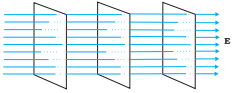Equipotential surfaces for (a) a dipole and (b) two identical positive charges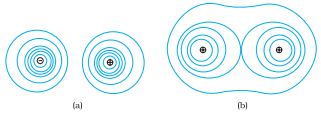## Electric Potential Energy

### Potential energy

The potential energy stored in a system of charges is the work done (by an external agency) in assembling the charges at their locations. The potential energy of two charges q1q2 at r1r2 is given by

where r12 is the distance between q1 and q2.

The potential energy of a charge in an external potential V(r) is qV(r). The potential energy of a dipole moment in a uniform electric field is –p.E.

### Electric Potential Energy

Consider an electrostatic field E due to a charge Q placed at the origin. Now, bring a test charge q from a point R to a point P against the repulsive force on it due to the charge Q. This will happen if Q and q are either positive or both negative.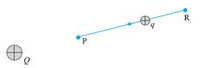Work done by external forces in moving a charge q from R to P is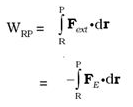At every point in the electric field, a particle with charge q possesses a certain electrostatic potential energy, this work done increases its potential energy by an amount equal to the potential energy difference between points R and P.

Thus, potential energy difference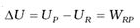(Note here that this displacement is in an opposite sense to the electric force and hence work done by electric field is negative, i.e., –WRP.)

Therefore, can define electric potential energy difference between two points as the work required to be done by an external force in moving (without accelerating) charge q from one point to another for the electric field of any arbitrary charge configuration.

• Work done by an electrostatic field in moving a charge from one point to another depends only on the initial and the final points and is independent of the path taken to go from one point to the other. This is the fundamental characteristic of a conservative force.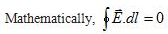• A convenient choice is to have electrostatic potential energy zero at infinity. With this choice, if take the point R at infinity,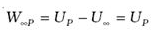The potential energy of charge q at a point (in the presence of field due to any charge configuration) is the work done by the external force (equal and opposite to the electric force) in bringing the charge q from infinity to that point.